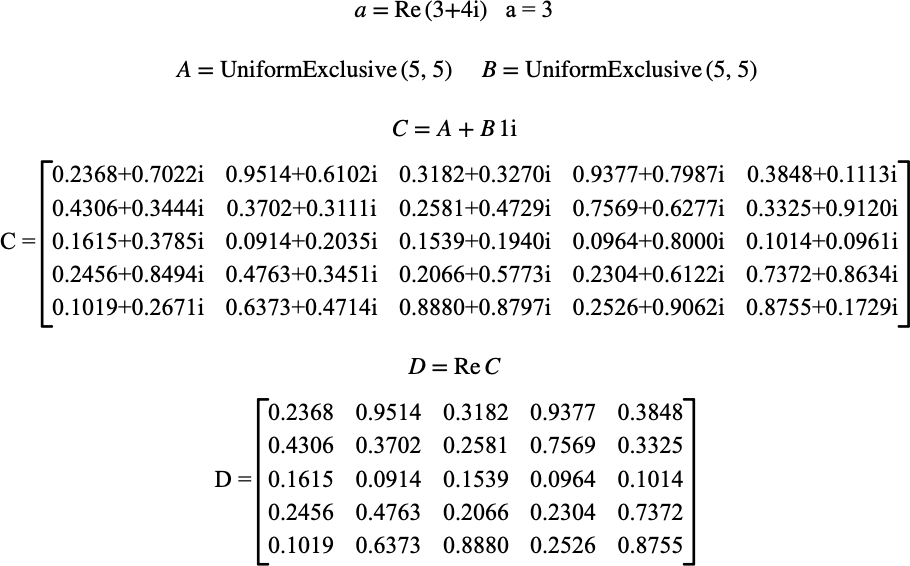# $$\text{Re}$$¶

You can use the $$\text{Re}$$ function to extract the real portion of a complex number. You can also use the $$\text{Re}$$ function to obtain a matrix containing the real portion of each element of a matrix.

You can use the \re backslash command to insert this function.

The following variants of this function are available:

• $$\text{scalar } \text{Re} \left ( \text{<value>} \right )$$

• $$\text{matrix } \text{Re} \left ( \text{<matrix>} \right )$$

The $$\text{<value>}$$ parameter can be any basic type. The $$\text{<matrix>}$$ parameter can be any matrix type.

The scalar version of the $$\text{Re}$$ function will return the most appropriate scalar type based on the supplied value. The matrix version of the $$\text{Re}$$ function will return the most appropriate matrix type based on the supplied matrix.

Aion will optimize the $$\text{Re}$$ function based on the supplied parameter allowing you to use this function on boolean, integer, and real types as well as boolean, integer, and real matrix types, if desired, with no computational overhead.

Figure 193 shows the basic use of the $$\text{Re}$$ function.Figure 193 Example Use Of the Re Function